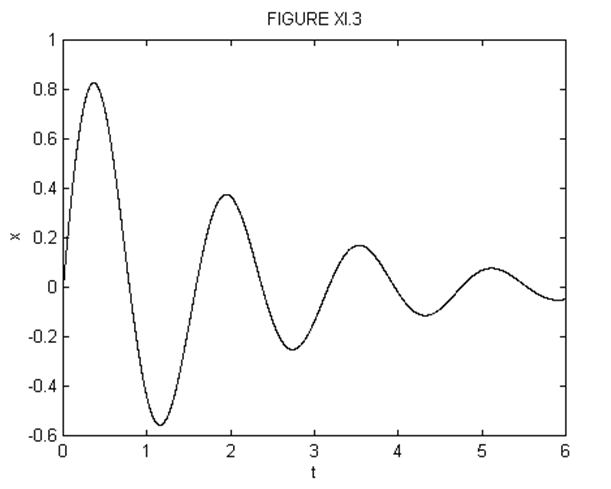$$\require{cancel}$$

# 11.5i: Light damping: $$\gamma < 2\omega_{0}$$

•• Contributed by Jeremy Tatum
• Emeritus Professor (Physics & Astronomy) at University of Victoria

Since $$\gamma < 2\omega_{0}$$, we have to write Equations 11.5.6 as

$k_{1}=-\frac{1}{2}\gamma+i\sqrt{\omega_{0}^{2}-\frac{1}{4}\gamma^{2}},\quad k_{2}=-\frac{1}{2}\gamma-i\sqrt{\omega_{0}^{2}-\frac{1}{4}\gamma^{2}}. \label{11.5.7}\tag{11.5.7}$

Further, I shall write

$\omega^{'}=\sqrt{w_{0}^{2}-\frac{1}{4}\gamma^{2}}. \label{11.5.8}\tag{11.5.8}$

Equation 11.5.4 is therefore

$x=Ae^{-\frac{1}{2}\gamma t+i\omega^{'}t}+Be^{-\frac{1}{2}\gamma t-i\omega^{'}t}=e^{-\frac{1}{2}\gamma t}(Ae^{+i\omega^{'}t}+Be^{-i\omega^{'}t}). \tag{11.5.9}\label{11.5.9}$

If $$x$$ is to be real, $$A$$ and $$B$$ must be complex. Also, since $$e^{-i\omega^{'}t}=(e^{i\omega^{'}t})$$* must equal $$A$$*, where the asterisk denotes the complex conjugate.

Let $$A=\frac{1}{2}(a-ib)$$ and $$B=\frac{1}{2}(a+ib)$$ where $$a$$ and $$b$$ are real. Then the reader should be able to show that Equation $$\ref{11.5.9}$$ can be written as

$x=e^{-\frac{1}{2}\gamma t}(a\cos\omega^{'}t+b\sin\omega^{'}t). \label{11.5.10}\tag{11.5.10}$

And if $$C=\sqrt{a^{2}+b^{2}}, \sin\alpha=\frac{a}{\sqrt{a^{2}+b^{2}}}, \cos\alpha=\frac{b}{\sqrt{a^{2}+b^{2}}},$$ the equation can be written

$x=Ce^{-\frac{1}{2}\gamma t}\sin(\omega^{'}t+\alpha). \label{11.5.11}\tag{11.5.11}$

Equations $$\ref{11.5.9}$$, $$\ref{11.5.10}$$ or $$\ref{11.5.11}$$ are three equivalent ways of writing the solution. Each has two arbitrary integration constants $$(A,B), (a,b)$$ or $$(C,\alpha)$$, whose values depend on the initial conditions - i.e. on the values of $$x$$ and $$\dot{x}$$ when $$t=0$$. Equation $$\ref{11.5.11}$$ shows that the motion is a sinusoidal oscillation of period a little less than $$\omega_{0}$$, with an exponentially decreasing amplitude.

To find $$C$$ and $$\alpha$$ in terms of the initial conditions, differentiate Equation $$\ref{11.5.11}$$ with respect to the time in order to obtain an equation showing the speed as a function of the time:

$\dot{x}=Ce^{-\frac{1}{2}\gamma t}[\omega^{'}\cos(\omega^{'}t+\alpha)-\frac{1}{2}\gamma\sin(\omega^{'}t+\alpha)]. \label{11.5.12}\tag{11.5.12}$

By putting $$t=0$$ in Equations $$\ref{11.5.11}$$ and $$\ref{11.5.12}$$ we obtain

$x_{0}=C\sin\alpha \label{11.5.13}\tag{11.5.13}$

and

$(\dot{x})_{0}=C(\omega^{'}\cos\alpha-\frac{1}{2}\gamma\sin\alpha). \label{11.5.14}\tag{11.5.14}$

From these we easily obtain

$\cot\alpha=\frac{1}{\omega^{'}}[\frac{(\dot{x})_{0}}{\dot{x}_{0}}+\frac{\gamma}{2}] \label{11.5.15}\tag{11.5.15}$

and

$C=x_{0}\csc\alpha. \label{11.5.16}\tag{11.5.16}$

The quadrant of $$\alpha$$ can be determined from the signs of $$\cot\alpha$$ and $$\csc\alpha$$ , $$C$$ always being positive.

Note that the amplitude of the motion falls off with time as $$e^{-\frac{1}{2}\gamma t}$$, but the mechanical energy, which is proportional to the square of the amplitude, falls off as $$e^{-\gamma t}$$.

Figures XI.2 and XI.3 are drawn for $$C=1, \alpha=0, \gamma=1$$. Figure XI.2 has $$\omega_{0}=25\gamma$$ and hence $$x=e^{-\frac{1}{2}t}\sin0.24.9949995t$$, and figure XI.3 has $$\omega_{0}=4\gamma$$ and hence $$x=e^{-\frac{1}{2}t}\sin3.968626967t$$.Exercise $$\PageIndex{1}$$

Draw displacement : time graphs for an oscillator with $$m$$ = 0.02 kg, $$k$$ = 0.08 N m-1, $$g$$ = 1.5 s-1, $$t$$ = 0 to 15 s, for the following initial conditions:

1. $$x_{0}=0,\quad (\dot{x})_{0}=4$$ ms-1
2. $$(\dot{x})_{0}=0,\quad x_{0}=3$$ m
3. $$(\dot{x})=-2$$ ms-1, $$x_{0}=2$$

Although the motion of a damped oscillator is not strictly "periodic", in that the motion does not repeat itself exactly, we could define a "period" $$P=\frac{2\pi}{\omega^{'}}$$ as the interval between two consecutive ascending zeroes. Extrema do not occur exactly halfway between consecutive zeroes, and the reader should have no difficulty in showing, by differentiation of Equation $$\ref{11.5.11}$$, that extrema occur at times given by $$\tan(\omega^{'}t+\alpha)=\frac{2\omega^{'}}{\gamma}$$. However, provided that the damping is not very large, consecutive extrema occur approximately at intervals of $$\frac{P}{2}$$. The ratio of consecutive maximum displacements is, then,

$\frac{|x_{n}|}{|x_{n+1}|}=\frac{e^{-\frac{1}{2}\gamma t}}{e^{-\frac{1}{2}\gamma (t+\frac{1}{2}P)}}. \label{11.5.17}\tag{11.5.17}$

From this, we find that the logarithmic decrement is

$ln(\frac{|x_{n}|}{|x_{n+1}|})=\frac{P\gamma}{4}, \label{11.5.18}\tag{11.5.18}$

from which the damping constant can be determined.